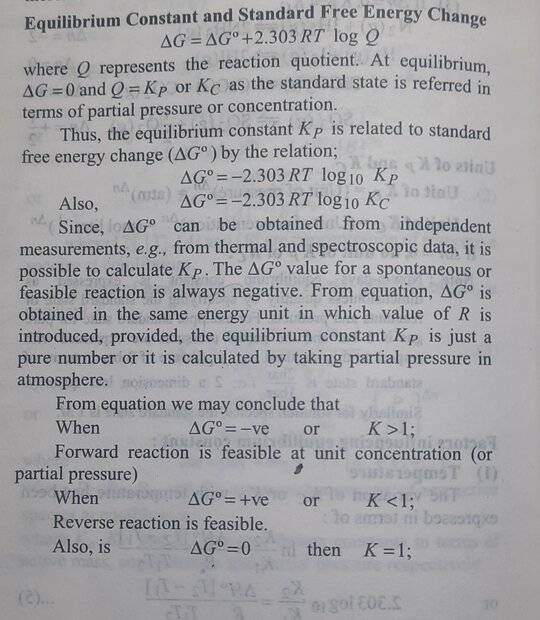# Confusion in relation of Gibbs free energy and equilibrium constant

• Chemistry
• tbn032
O2(g) and you want to know the equilibrium constant for the reaction. You would use the equation Keq=Kp.

#### tbn032

Homework Statement
for the reaction
SO2(g)+(1/2)O2(g)⇌SO3(g);ΔH=-98.32KJ/mole,ΔS=-95J/(mole-K).
find Kp at 298 Kelvin?
Relevant Equations
SO2(g)+(1/2)O2(g)⇌SO3(g);ΔH=-98.32KJ/mole,ΔS=-95J/(mole-K).
SO2(g)+1/2O2(g)SO3(g);ΔHo=-98.32KJ/mole,ΔSo=-95J/(mole-K).
find Kp at 298 Kelvin?
In given question at first Δ G will be calculated using formula ΔG = Δ H – T x ΔS, by putting the given values in formula we get ΔG = -70.01 kJ/mol.
Then Keq will be calculated using equation = Δ G = -RT ln Keq ,ln Keq = -70010J/-8.314 x 298 = 28.25 .or Keq = e28.25 = 1.86x1012.
Now my confusion is Keq=Kpor Keq=Kc(depending on if we measure the equilibrium constant in terms of pressure or molarity, gases can be measured in both).and
Kp = Kc(RT)Δn and Δn=-1/2(for this reaction).
No information about the pressure or concentration is given so how do I conclude that Keq=Kp or Keq=Kc and thus calculate the correct value of Kp

Last edited:
Given the temperature 298 Kelvin, is this reaction at the 'standard state'? If so, the pressure is ambient or ~1 atm (100 kPa, or 1 bar).
https://en.wikipedia.org/wiki/Standard_state#Conventional_standard_states

Given the standard state, one should be able to determine the density of the gases. What does one's textbook indicate about STP, standard state and Gibbs free energy?

The problem statement one provides indicates gases.

Last edited:
my confusion is arising from the fact that in my book it is written that
Δ G = -RT ln Keq
Keq=KporKc as the standard state is referred in terms of partial pressure or concentration
Δ G = -RT ln Kp
Δ G = -RT ln Kk
if calculate the value of Keq from the equation Δ G = -RT ln Keq using R=8.3144598J⋅K-1⋅mol-1.the value of Keq is dimensionless
so I am not able to conclude if Keq=Kp or Keq=Kc
kp≠kc because Kp = Kc(RT)Δn and Δn=-1/2(for this reaction).Last edited:
The standard state for gases is 1 bar. So, in this example, the Keq is Kp. It is in terms of the partial pressures of the components in bars.

Incidentally, you omitted the superscript 0 in ##\Delta G^0##

•Lord Jestocost
tbn032 said:
Homework Statement:: for the reaction
SO2(g)+(1/2)O2(g)⇌SO3(g);ΔH=-98.32KJ/mole,ΔS=-95J/(mole-K).
find Kp at 298 Kelvin?
Relevant Equations:: SO2(g)+(1/2)O2(g)⇌SO3(g);ΔH=-98.32KJ/mole,ΔS=-95J/(mole-K).

SO2(g)+1/2O2(g)SO3(g);ΔHo=-98.32KJ/mole,ΔSo=-95J/(mole-K).
find Kp at 298 Kelvin?
In given question at first Δ G will be calculated using formula ΔG = Δ H – T x ΔS, by putting the given values in formula we get ΔG = -70.01 kJ/mol.
Then Keq will be calculated using equation = Δ G = -RT ln Keq ,ln Keq = -70010J/-8.314 x 298 = 28.25 .or Keq = e28.25 = 1.86x1012.
Now my confusion is Keq=Kpor Keq=Kc(depending on if we measure the equilibrium constant in terms of pressure or molarity, gases can be measured in both).and
Kp = Kc(RT)Δn and Δn=-1/2(for this reaction).
No information about the pressure or concentration is given so how do I conclude that Keq=Kp or Keq=Kc and thus calculate the correct value of Kp
The equation is giving it up as you have SO2(g) you are dealing with gases